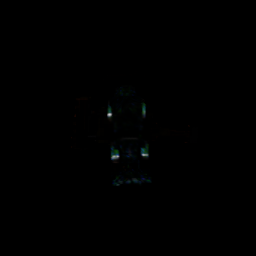# Bug: Some writer RGB images are blacked out when generating with randomizer.instantiate

Hi,

I’m working in headless mode to generate RGB images and Semantic Masks for several models (usd files all under the same directory), for each frame, I want only a single object with its mask.

To do that, I’m using randomizer.instantiate with size=1, after calling utils.get_usd_files on the models directory (this is similar to the SGD tutorial).

For most results, this is working correctly, however some images (about 5%) are completely blacked outThe segmentation mask is still fine.
Note the source USD file is ok - If I’m only running the script on a single model at a time (using the from_usd command) this works well with all models in the folder.

Here’s the code:

``````with rep.new_layer():
if not os.path.isdir(PROPS):
camera_pos = (0, 2000, 0)
camera = rep.create.camera(position=camera_pos, look_at=(0, 0, 0))
render_product = rep.create.render_product(camera, IMAGE_SIZE)
scene_light = rep.create.light(
light_type="Distant",
temperature=6500,
intensity=500,
rotation=(315, 0, 0),
count=1
)

props = rep.utils.get_usd_files(PROPS, recursive=True)
with rep.trigger.on_frame(num_frames=FRAMES):
object_instance = rep.randomizer.instantiate(props, size=1, mode='scene_instance')
with object_instance:
rep.modify.semantics([('class', 'object')])
rep.modify.pose(rotation=rep.distribution.uniform((0, -180, 0), (0, 180, 0)), position=(0, 0, 1.2))

writer = rep.WriterRegistry.get("BasicWriter")
writer.initialize(
output_dir=output_dir,
rgb=True,
semantic_segmentation=True,
)
writer.attach([render_product])

rep.orchestrator.run()`````````